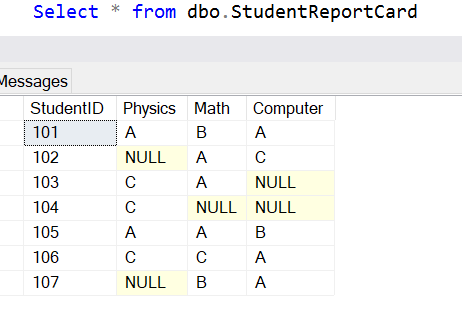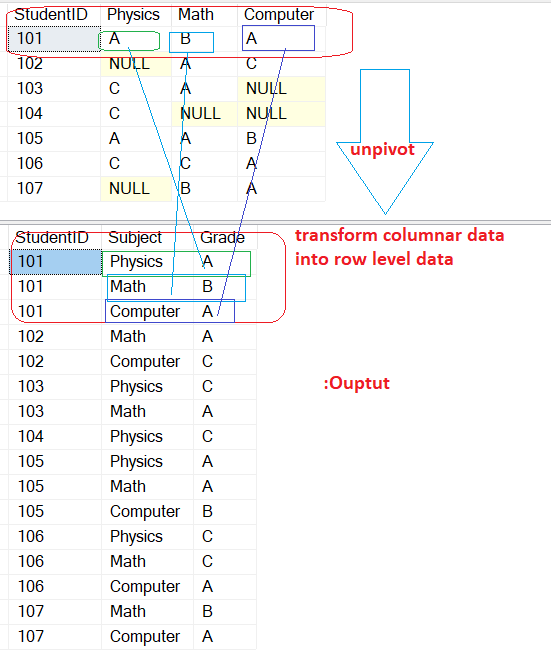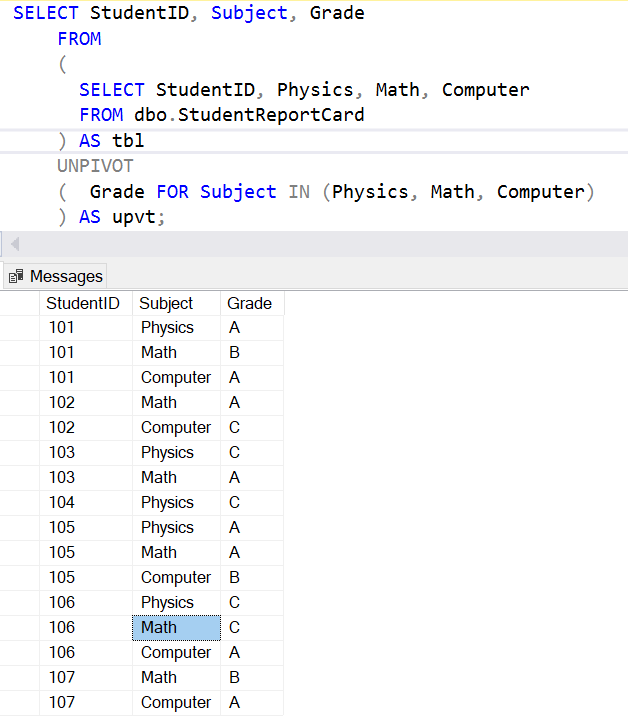Home » SQL Server UNPIVOT

# SQL Server UNPIVOTSQL Server UNPIVOT allow us to transform columns of a table-valued expression into column values that is rotating columns into rows.

It performs a reverse operation of SQL Server PIVOT.

SYNTAX

```
SELECT ...
FROM ...
UNPIVOT ( <value_column>
FOR <pivot_column> IN ( <column_list> ) )

[ ... ]```

pivot_column contains the column values that you want to rotate.

value column  holds the values that currently exist under the columns being rotated.

Column_list  it contains columns name , it should not be any subquery.

Lets look at an example of SQL Server unpivot, Here we have created a sample table of StudentReportCard.

```
CREATE TABLE dbo.StudentReportCard

(
StudentID INT PRIMARY KEY,
Physics CHAR(3),
Math CHAR(3),
Computer CHAR(3)

);
INSERT dbo.StudentReportCard VALUES
(101,'A', 'B', 'A'),
(102, NULL, 'A', 'C' ),
(103, 'C', 'A', NULL ),
(104, 'C', NULL, NULL ),
(105, 'A', 'A', 'B' ),
(106, 'C', 'C', 'A' ),
(107, NULL, 'B', 'A' )```Lets quickly check a table records.

`Select * from dbo.StudentReportCard`Now we have a StudentReportCard table which contains a student’s performance in terms of grade in three subjects as showing in a table columns: Physics , Math, Computer.

Now we want to transform these column into row values correspond to a particular student as shown in below given screenshot.To achieve the expected output you need to specify two additional columns .

First column (pivot column) will contain the column values that you are rotating as Physics, Math and computer will be called as Subject, and the second column (value column) that will hold the values that currently exist under the columns being rotated will be called as Grade.

```SELECT StudentID, Subject, Grade
FROM
(
SELECT StudentID, Physics, Math, Computer
FROM dbo.StudentReportCard
) AS tbl
UNPIVOT
(

Grade FOR Subject IN (Physics, Math, Computer)

) AS upvt;```

As you can see, multiple columns (physics, math and computer) are transformed into single column as subject and their value is displayed into column Grade.